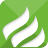# es6-解构赋值用途

2018-07-10 09:49:51 | 浏览量：343 前沿信息 es6

开发中经常定义许多对象和数组，然后有根据需要从中提取相关的信息片段。在ES6中添加了可以简化这种任务的新特性：解构。解构是一种打破数据结构，将其拆分为更小部分的过程。

```let options = { width: 500, height: 300 };

// 从对象中提取数据
let width = options.width, height = options.height;```

这段代码从options对象中提取width和height的值，并将其存储为同名局部变量，如果要提取更多变量，则必须依次编写类似的代码来为变量赋值，如果其中还包含嵌套结构，只靠遍历是找不到真实信息的，必须要深入挖掘整个数据结构才能找到所需数据,那将更麻烦了。

`let { width, height } = options;`

## 数组的解构赋值

`let firstColor = "red"，secondColor = "gren" ,thirdColor = "blue";`

ES6 允许的写法：

```let colors = [ "red", "green" ];
let [ firstColor, secondColor ] = colors;
console.log(firstColor); // "red"
console.log(secondColor); // "green"```

`console.log(thirdColor);//undefined`

```let colors = [ "red", "green", "blue" ];
let [ , , thirdColor ] = colors;
console.log(thirdColor); // "blue"```

【默认值】

```let colors = [ "red" ];
let [ firstColor, secondColor = "green" ] = colors;
console.log(firstColor); // "red"
console.log(secondColor); // "green"
```

【嵌套数组解构】

```let colors = [ "red", [ "green", "lightgreen" ], "blue" ];
let [ firstColor, [ secondColor ] ] = colors;
console.log(firstColor); // "red"
console.log(secondColor); // "green"```

【不定元素】

```let colors = [ "red", "green", "blue" ];
let [ firstColor, ...restColors ] = colors;
console.log(firstColor); // "red"
console.log(restColors.length); // 2
console.log(restColors); // "green"
console.log(restColors); // "blue"```

[注意]在被解构的数组中，不定元素必须为最后一个条目，在后面继续添加逗号会导致程序抛出语法错误。

【数组复制】

```var colors = [ "red", "green", "blue" ];
var clonedColors = colors.concat();
console.log(clonedColors); //"[red,green,blue]"```

concat()方法的设计初衷是连接两个数组，如果调用时不传递参数就会返回当前函数的副本。

```let colors = [ "red", "green", "blue" ];
let [ ...clonedColors ] = colors;
console.log(clonedColors); //"[red,green,blue]"```

## 对象的解构赋值

```let node = {
type: "Identifier",
name: "foo"
};

let { type, name } = node;
console.log(type); // "Identifier"
console.log(name); // "foo"```

【解构赋值】

```let node = {
type: "Identifier",
name: "foo"
},

type = "Literal",
name = 5;
//使用解构来分配不同的值
({ type, name } = node);
console.log(type); // "Identifier"
console.log(name); // "foo"```

[注意]一定要用一对小括号包裹解构赋值语句，JS引擎将一对开放的花括号视为一个代码块。语法规定，代码块语句不允许出现在赋值语句左侧，添加小括号后可以将块语句转化为一个表达式，从而实现整个解构赋值过程。

`var {x = 3} = {}; // x=3`

【为非同名局部变量赋值】

```let node = {
type: "Identifier",
name: "foo"
};

let { type: localType, name: localName } = node;
console.log(localType); // "Identifier"
console.log(localName); // "foo"
console.log(type) // error: type is not defined```

当使用其他变量名进行赋值时也可以添加默认值，只需在变量名后添加等号和默认值即可

```let node = {
type: "Identifier"
};

let { type: localType, name: localName = "bar" } = node;
console.log(localType); // "Identifier"
console.log(localName); // "bar"```

【嵌套对象解构】

```let node = {
type: "Identifier",
name: "foo",
loc: {
start: {
line: 1,
column: 1
},
end: {
line: 1,
column: 4
}
}
};

let { loc: { start }} = node;
console.log(start.line); // 1
console.log(start.column); // 1```

```let node = {
type: "Identifier",
name: "foo",
loc: {
start: {
line: 1,
column: 1
},
end: {
line: 1,
column: 4
}
}
};

//提取node.loc.start
let { loc: { start: localStart }} = node;
console.log(localStart.line); // 1
console.log(localStart.column); // 1```

```function add([x, y]){
return x + y;
}
add([1, 2]); // 3```

```function move({x, y} = { x: 0, y: 0 }) {
return [x, y];
}
move({x: 3, y: 8}); // [3, 8]
move({x: 3}); // [3, undefined]
move({}); // [undefined, undefined]
move(); // [0, 0]```

## 混合解构

```let node = {
type: "Identifier",
name: "foo",
loc: {
start: {
line: 1,
column: 1
},
end: {
line: 1,
column: 4
}
},
range: [0, 3]
};
let {
loc: { start },
range: [ startIndex ]
} = node;
console.log(start.line); // 1
console.log(start.column); // 1
console.log(startIndex); // 0```

## 其他用途

1）交换变量的值：

```let a = 1, b = 2, tmp;
tmp = a;
a = b;
b = tmp;
console.log(a); // 2
console.log(b); // 1```

```let a = 1,
b = 2;
[ a, b ] = [ b, a ];
console.log(a); // 2
console.log(b); // 1```

（2）从函数返回多个值

//返回一个数组

```function example() {
return [1, 2, 3];
}
let [a, b, c] = example();
//返回一个对象
function example() {
return {
foo: 1,
bar: 2
};
}
let { foo, bar } = example();```

3）函数参数的定义

//参数是一组有次序的值

```function f([x, y, z]) { ... }
f([1, 2, 3]);```

//参数是一组无次序的值

```function f({x, y, z}) { ... }
f({z: 3, y: 2, x: 1});```

（4）提取JSON数据

```let jsonData = {
id: 42,status: "OK",data: [867, 5309]
};
let { id, status, data: number } = jsonData;
console.log(id, status, number);//42, "OK", [867, 5309]```

（5）函数参数的默认值

```jQuery.ajax = function (url, {
async = true,
beforeSend = function () {},
cache = true,
complete = function () {},
crossDomain = false,
global = true,
//...more config
}) {
//...do stuff
};```

（6）遍历Map结构

```const map = new Map();
map.set('first', 'hello');
map.set('second', 'world');
for (let [key, value] of map) {
console.log(key + " is " + value);
}
//first is hello
//second is world```

//获取键名

```for (let [key] of map) {
// ...
}```

//获取键值

```for (let [,value] of map) {
// ...
}```
（7）输入模块的指定方法

`const { SourceMapConsumer, SourceNode } = require("source-map");`

## 其他字符串的解构赋值

```const [a, b, c, d, e] = 'hello';
//a= "h"，b= "e",c= "l",d="l",e="o"```

`let {length : len} = 'hello'; // len=5`

【数值和布尔值解构】

```let {toString:s1} = 123;
console.log(s1 === Number.prototype.toString);//true
let {toString:s2} = true;
console.log(s2 === Boolean.prototype.toString);//true```

```let { prop: x } = undefined; // TypeError
let { prop: y } = null; // TypeError```

0.7397s